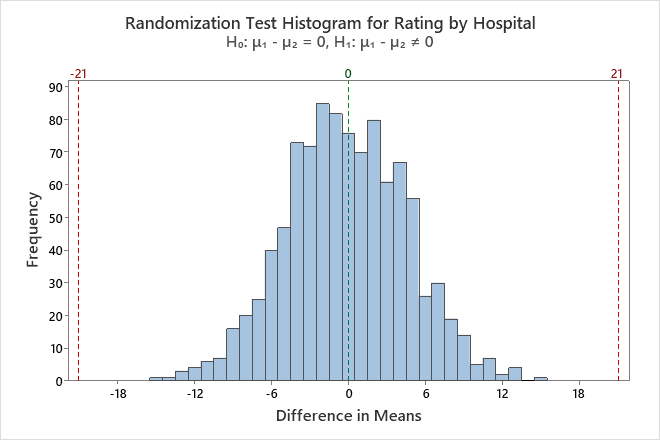# Interpret the key results for Randomization test for 2-sample means

Complete the following steps to interpret a randomization test for 2-sample means. Key output includes the histogram and the p-value.

## Step 1: Examine the shape of your bootstrap distribution

Use the histogram to examine the shape of your bootstrap distribution. The bootstrap distribution is the distribution of the chosen statistic from each resample. The bootstrap distribution should appear to be normal. If the bootstrap distribution is non-normal, you cannot trust the bootstrap results.The distribution is usually easier to determine with more resamples. For example, in these data, the distribution is ambiguous for 50 resamples. With 1000 resamples, the shape looks approximately normal.In this histogram, the bootstrap distribution appears to be normal.

## Step 2: Determine whether the test results are statistically significant

To determine whether the difference between population means is statistically significant, compare the p-value to the significance level. Usually, a significance level (denoted as α or alpha) of 0.05 works well. A significance level of 0.05 indicates a 5% risk of concluding that a difference exists when there is no actual difference.
P-value ≤ α: The difference between the means is statistically significant (Reject H0)
If the p-value is less than or equal to the significance level, the decision is to reject the null hypothesis. You can conclude that the difference between the population means is statistically significant. To calculate a confidence interval and determine whether the difference is practically significant, use Bootstrapping for 2-sample means. For more information, go to Statistical and practical significance.
P-value > α: The difference between the means is not statistically significant (Fail to reject H0)
If the p-value is greater than the significance level, the decision is to fail to reject the null hypothesis. You do not have enough evidence to conclude that the difference between the population means is statistically significant.
By using this site you agree to the use of cookies for analytics and personalized content.  Read our policy